## Metaphysics on geometric distribution in probability theory

I realized geometric distribution is not exactly about the time needed to get the first success in a given number of trials. This is a very odd feeling. It is probably a feeling applied mathematicians get sometimes, when they feel they are doing the best they can, and yet the theory is not perfect.

This may be a naive post, I warn you, but I was really stunned when I realized this.

### Geometric distribution is not about the first success

Let’s jump to the point. We know (or at least, I was taught) that geometric distribution is used to calculate the probability that the first success in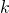trials (all independent and of probability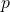) will happen precisely at the-th trial.

Remember that a geometric distribution is a random variable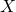such that its distribution is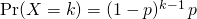How can we relate the above distribution with the fact that it matches the first success? Well, we need to have one success, which explains theat the bottom. Moreover, we want to have just one success, so all other trials must be unsuccessful, which explains the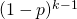.

But hey, where would first ever be written? Continue reading “Metaphysics on geometric distribution in probability theory”